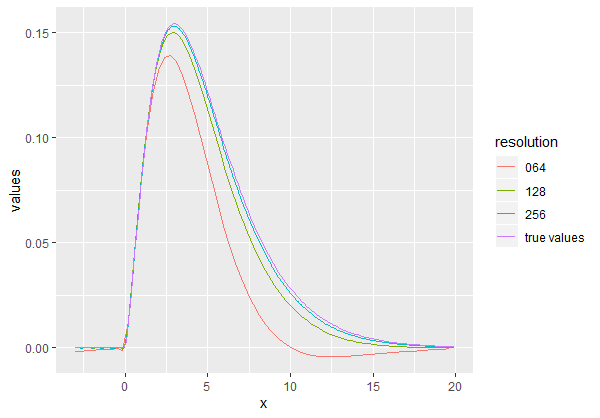# mathclasstutor

Mathematics, Science, statistics, economics, finance, econometrics, Business Mathematics, statistical analysis, R language, Computer Science, Stock Analysis, APPLE Stock Analysis, Amazon stock Analysis, GARCH model, Arch model,q-q plot,ols, regression, Time Series, t-test, cofactor, variance, standard deviation,Matlab,Nifty 50 Analysis,BMW stock analysis,

## Email Subscribers

Delivered by FeedBurner

## Relative strength index (RSI)NSEI

RSI is one of the most popular technical indicators in the stock market. It is computed based on the speed and direction of the stock price movement.RSI is calculated for the two-step process. First, the average gains and losses are identified for a specified time period. You can consider any time period but 14 days RSI is commonly used. Suppose that the stock went off on nine days and fail on five days. The absolute stock price gain on each of these nine days are are added up and divided by 14 to get the average gains. Similarly, the absolute loss on each of the five days are added up and divided by 14 to get the average losses. The ratio between these values is known as relative strength.
Second, the indicator is normalized later to make sure that RSI always moves between 0 and 100 the value will be 0 if the stock fell in all the 14 days and will be 100 if the price move of on all the 14 days.
Formula
RSI=100-[100/(1+RS)].
Oversold and overbought levels
RSI value is designed to move between zero and hundred always it can be used to identify the overbought and oversold level in a counter above 70 as an overbought zone and below 30 age oversold.